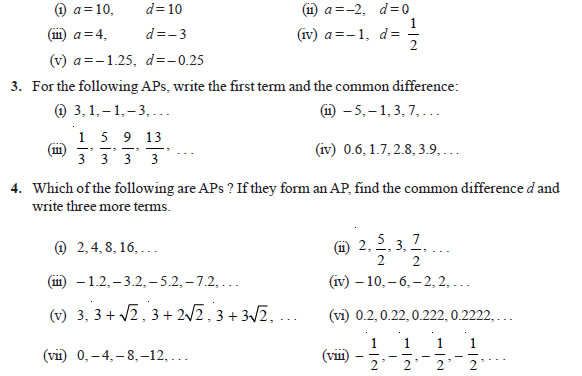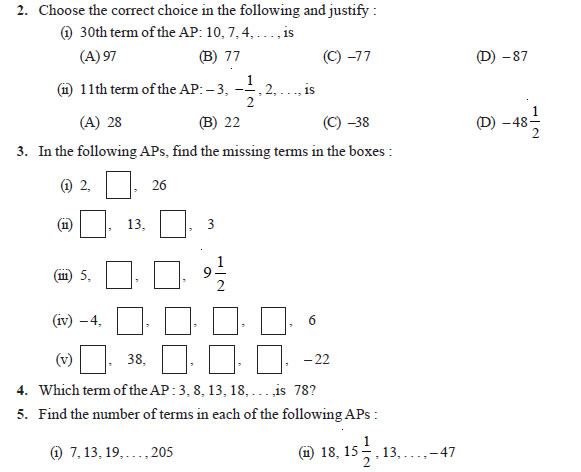# Arithmetic Progressions Class 10 NCERT Solutions

NCERT Solutions Class 10 Mathematics Chapter 5 Arithmetic Progressions Download In Pdf

## In this pdf file you can see answers of following Questions

### EXERCISE 5.1

Question 1. In which of the following situations, does the list of numbers involved make an arithmetic progression, and why?
(i) The taxi fare after each km when the fare is Rs 15 for the first km and Rs 8 for each additional km.
(ii) The amount of air present in a cylinder when a vacuum pump removes 1 4 of the air remaining in the cylinder at a time.
(iii) The cost of digging a well after every metre of digging, when it costs Rs 150 for the first metre and rises by Rs 50 for each subsequent metre.
(iv) The amount of money in the account every year, when Rs 10000 is deposited at compound interest at 8 % per annum.

Question 2. Write first four terms of the AP, when the first term a and the common difference d are given as follows:### EXERCISE 5.2

Question 1. Fill in the blanks in the following table, given that a is the first term, d the common difference and an the nth term of the AP:Question 6. Check whether – 150 is a term of the AP : 11, 8, 5, 2 . . .

Question 7. Find the 31st term of an AP whose 11th term is 38 and the 16th term is 73.

Question 8. An AP consists of 50 terms of which 3rd term is 12 and the last term is 106. Find the 29th term.

Question 9. If the 3rd and the 9th terms of an AP are 4 and – 8 respectively, which term of this AP is zero?

Question 10. The 17th term of an AP exceeds its 10th term by 7. Find the common difference.

Question 11. Which term of the AP : 3, 15, 27, 39, . . . will be 132 more than its 54th term?

Question 12. Two APs have the same common difference. The difference between their 100th terms is 100, what is the difference between their 1000th terms?

Question 13. How many three-digit numbers are divisible by 7?

Question 14. How many multiples of 4 lie between 10 and 250?

Question 15. For what value of n, are the nth terms of two APs: 63, 65, 67, . . . and 3, 10, 17, . . . equal? 16. Determine the AP whose third term is 16 and the 7th term exceeds the 5th term by 12.

### EXERCISE 5.3

Question 1. Find the sum of the following APs: (i) 2, 7, 12, . . ., to 10 terms.
(ii) –37, –33, –29, . . ., to 12 terms.
(iii) 0.6, 1.7, 2.8, . . ., to 100 terms.
(iv) 1 , 1 , 1 15 12 10 , . . ., to 11 terms.

Question 2. Find the sums given below :
(i) 7 + 10 1 2 + 14 + . . . + 84
(ii) 34 + 32 + 30 + . . . + 10
(iii) –5 + (–8) + (–11) + . . . + (–230) 3. In an AP:
(i) given a = 5, d = 3, an = 50, find n and Sn.
(ii) given a = 7, a13 = 35, find d and S13.
(iii) given a12 = 37, d = 3, find a and S12.
(iv) given a3 = 15, S10 = 125, find d and a10.
(v) given d = 5, S9 = 75, find a and a9. (vi) given a = 2, d = 8, Sn = 90, find n and an.
(vii) given a = 8, an = 62, Sn = 210, find n and d.
(viii) given an = 4, d = 2, Sn = –14, find n and a.
(ix) given a = 3, n = 8, S = 192, find d. (x) given l = 28, S = 144, and there are total 9 terms. Find a

Question 4. How many terms of the AP : 9, 17, 25, . . . must be taken to give a sum of 636?

Question 5. The first term of an AP is 5, the last term is 45 and the sum is 400. Find the number of terms and the common difference.

Question 6. The first and the last terms of an AP are 17 and 350 respectively. If the common difference is 9, how many terms are there and what is their sum?

Question 7. Find the sum of first 22 terms of an AP in which d = 7 and 22nd term is 149.

Question 8. Find the sum of first 51 terms of an AP whose second and third terms are 14 and 18 respectively.

Question 9. If the sum of first 7 terms of an AP is 49 and that of 17 terms is 289, find the sum of first n terms.

Question 10. Show that a1, a2, . . ., an, . . . form an AP where an is defined as below :
(i) an = 3 + 4n
(ii) an = 9 – 5n Also find the sum of the first 15 terms in each case.

Question 11. If the sum of the first n terms of an AP is 4n – n2, what is the first term (that is S1)? What is the sum of first two terms? What is the second term? Similarly, find the 3rd, the 10th and the nth terms.

Question 12. Find the sum of the first 40 positive integers divisible by 6.

Question 13. Find the sum of the first 15 multiples of 8.

Question 14. Find the sum of the odd numbers between 0 and 50.

Question 15. A contract on construction job specifies a penalty for delay of completion beyond a certain date as follows: Rs 200 for the first day, Rs 250 for the second day, Rs 300 for the third day, etc., the penalty for each succeeding day being Rs 50 more than for the preceding day. How much money the contractor has to pay as penalty, if he has delayed the work by 30 days?

Question 16. A sum of Rs 700 is to be used to give seven cash prizes to students of a school for their overall academic performance. If each prize is Rs 20 less than its preceding prize, find the value of each of the prizes.

Question 17. In a school, students thought of planting trees in and around the school to reduce air pollution. It was decided that the number of trees, that each section of each class will plant, will be the same as the class, in which they are studying, e.g., a section of Class I will plant 1 tree, a section of Class II will plant 2 trees and so on till Class XII. There are three sections of each class. How many trees will be planted by the students?

Question 18. A spiral is made up of successive semicircles, with centres alternately at A and B, starting with centre at A, of radii 0.5 cm, 1.0 cm, 1.5 cm, 2.0 cm, . . . as shown in Fig. 5.4. What is the total length of such a spiral made up of thirteen consecutive semicircles? (Take π = 22 7 ) 114 MATHEMATICS Fig. 5.4 [Hint : Length of successive semicircles is l1, l2, l3, l4, . . . with centres at A, B, A, B, . . ., respectively.]

Question 19. 200 logs are stacked in the following manner: 20 logs in the bottom row, 19 in the next row, 18 in the row next to it and so on (see Fig. 5.5). In how may rows are the 200 logs placed and how many logs are in the top row? Fig. 5.5

Question 20. In a potato race, a bucket is placed at the starting point, which is 5 m from the first potato, and the other potatoes are placed 3 m apart in a straight line. There are ten potatoes in the line (see Fig. 5.6). Fig. 5.6 A competitor starts from the bucket, picks up the nearest potato, runs back with it, drops it in the bucket, runs back to pick up the next potato, runs to the bucket to drop it in, and she continues in the same way until all the potatoes are in the bucket. What is the total distance the competitor has to run? [Hint : To pick up the first potato and the second potato, the total distance (in metres) run by a competitor is 2 × 5 + 2 × (5 + 3)]

#### EXERCISE 5.4 (Optional)*

Question 1. Which term of the AP : 121, 117, 113, . . ., is its first negative term? [Hint : Find n for an < 0]

Question 2. The sum of the third and the seventh terms of an AP is 6 and their product is 8. Find the sum of first sixteen terms of the AP.

Question 3. A ladder has rungs 25 cm apart. (see Fig. 5.7). The rungs decrease uniformly in length from 45 cm at the bottom to 25 cm at the top. If the top and the bottom rungs are 2 1 2 m apart, what is the length of the wood required for the rungs? [Hint : Number of rungs = 250 25 ]

Question 4. The houses of a row are numbered consecutively from 1 to 49. Show that there is a value of x such that the sum of the numbers of the houses preceding the house numbered x is equal to the sum of the numbers of the houses following it. Find this value of x. [Hint : Sx – 1 = S49 – Sx]

Question 5. A small terrace at a football ground comprises of 15 steps each of which is 50 m long and built of solid concrete. Each step has a rise of 1 4 m and a tread of 1 2 m. (see Fig. 5.8). Calculate the total volume of concrete required to build the terrace. [Hint : Volume of concrete required to build the first step = 1 1 50 m3 4 2 × × ]### NCERT Books Free Pdf Download for Class 5, 6, 7, 8, 9, 10 , 11, 12 Hindi and English Medium

 Mathematics Biology Psychology Chemistry English Economics Sociology Hindi Business Studies Geography Science Political Science Statistics Physics Accountancy